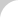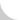The Complete Guide to Chain# 4.2.8 Calculation of Tension

Here we have an example to determine the tension in a horizontal conveyor and free flow conveyor.

Terms

• Tmax: Maximum chain tension (kN).
• T: Static chain tension at each part of conveyor (kN).
• Q: Maximum weight of conveyed objects (t/h).
• V: Conveying speed (the chain speed). (m/min.).
• H: Vertical center distance between sprockets (m).
• L: Horizontal center distance between sprockets (m).
• C: Center distance between sprockets (m).
• m: Mass of the working portion of the chain (kg/m). The mass of the chain x number of the chain strands, bucket, apron, etc.
• M: Mass of the conveyed object in conveying section (kg/m).
• f1: Coefficient of friction between the chain and the guide rail when conveying.
• f2: Coefficient of friction between the chain and the conveyed objects in the accumulating section.